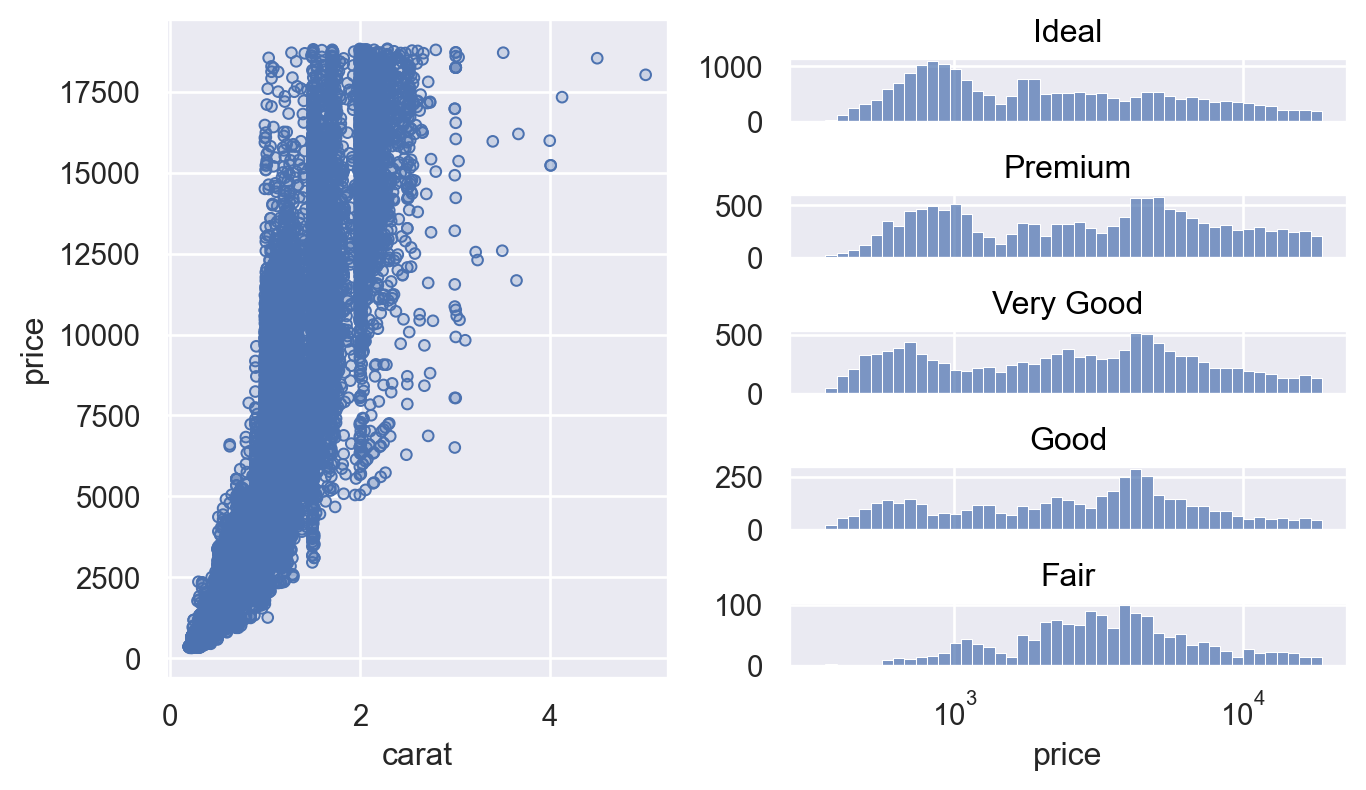# seaborn.objects.Plot.on#

Plot.on(target)#

Provide existing Matplotlib figure or axes for drawing the plot.

When using this method, you will also need to explicitly call a method that triggers compilation, such as `Plot.show()` or `Plot.save()`. If you want to postprocess using matplotlib, you’d need to call `Plot.plot()` first to compile the plot without rendering it.

Parameters:
targetAxes, SubFigure, or Figure

Matplotlib object to use. Passing `matplotlib.axes.Axes` will add artists without otherwise modifying the figure. Otherwise, subplots will be created within the space of the given `matplotlib.figure.Figure` or `matplotlib.figure.SubFigure`.

Examples

Passing a `matplotlib.axes.Axes` object provides functionality closest to seaborn’s axes-level plotting functions. Notice how the resulting image looks different from others created with `Plot`. This is because the plot theme uses the global rcParams at the time the axes were created, rather than `Plot` defaults:

```p = so.Plot(diamonds, "carat", "price").add(so.Dots())
f, ax = plt.subplots()
p.on(ax).show()
```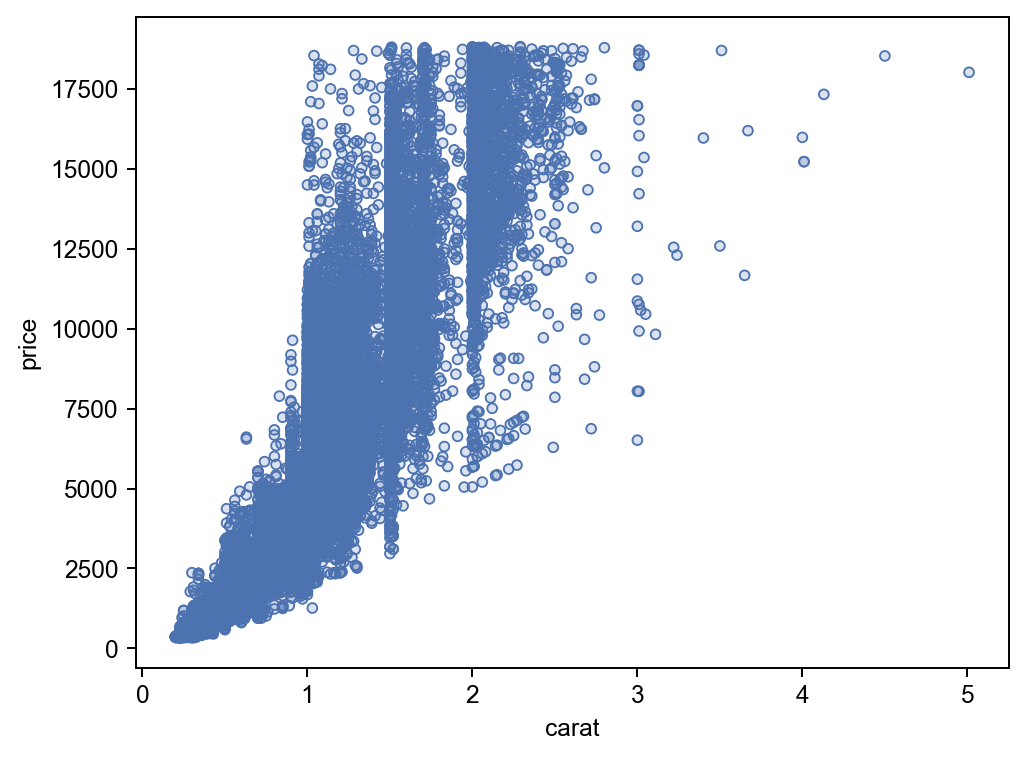Alternatively, calling `matplotlib.pyplot.figure()` will defer axes creation to `Plot`, which will apply the default theme (and any customizations specified with `Plot.theme()`):

```f = plt.figure()
p.on(f).show()
```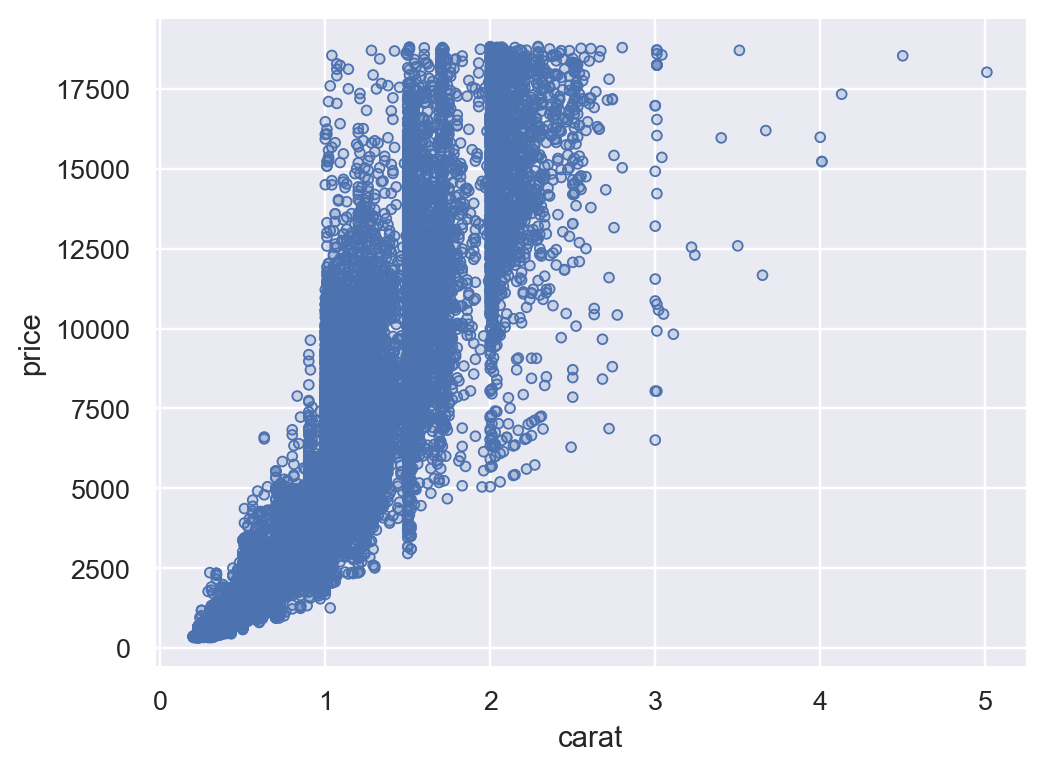Creating a `matplotlib.figure.Figure` object will bypass `pyplot` altogether. This may be useful for embedding `Plot` figures in a GUI application:

```f = mpl.figure.Figure()
p.on(f).plot()
```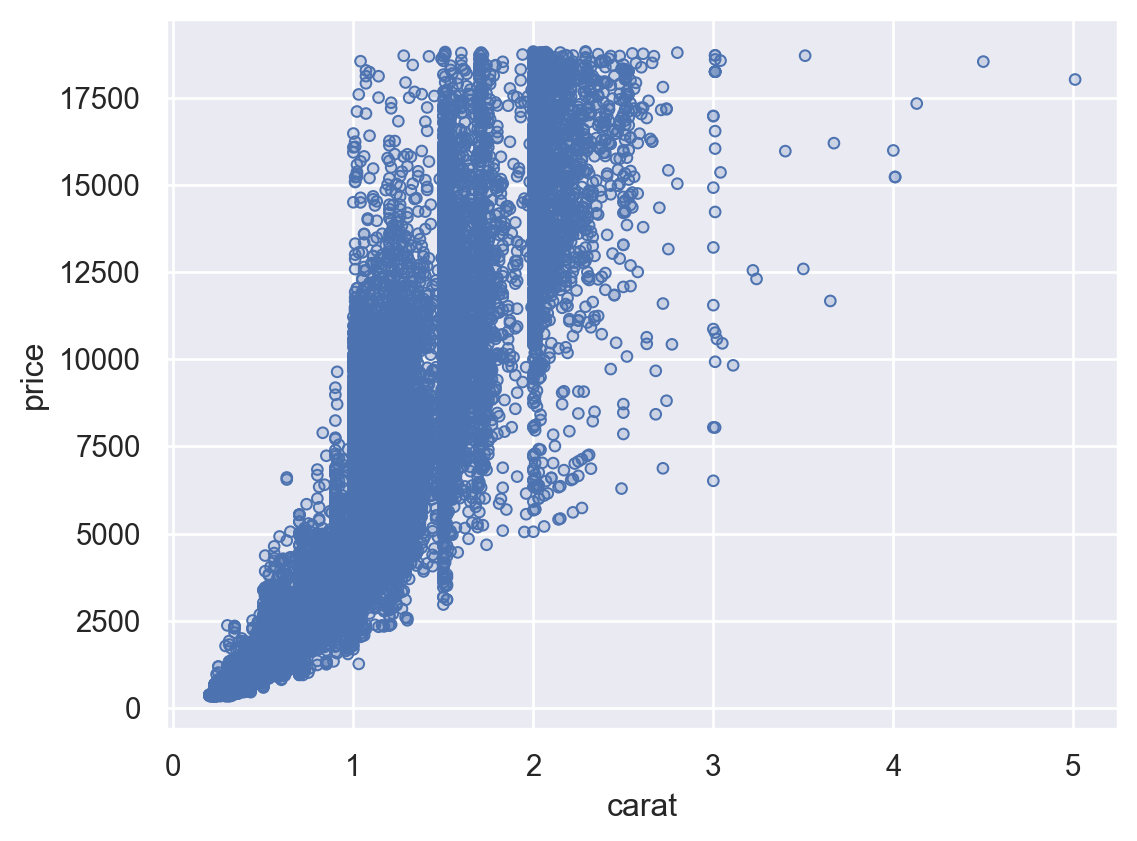Using `Plot.on` also provides access to the underlying matplotlib objects, which may be useful for deep customization. But it requires a careful attention to the order of operations by which the `Plot` is specified, compiled, customized, and displayed:

```f = mpl.figure.Figure()
res = p.on(f).plot()

ax = f.axes
rect = mpl.patches.Rectangle(
xy=(0, 1), width=.4, height=.1,
color="C1", alpha=.2,
transform=ax.transAxes, clip_on=False,
)
ax.text(
x=rect.get_width() / 2, y=1 + rect.get_height() / 2,
s="Diamonds: very sparkly!", size=12,
ha="center", va="center", transform=ax.transAxes,
)

res
```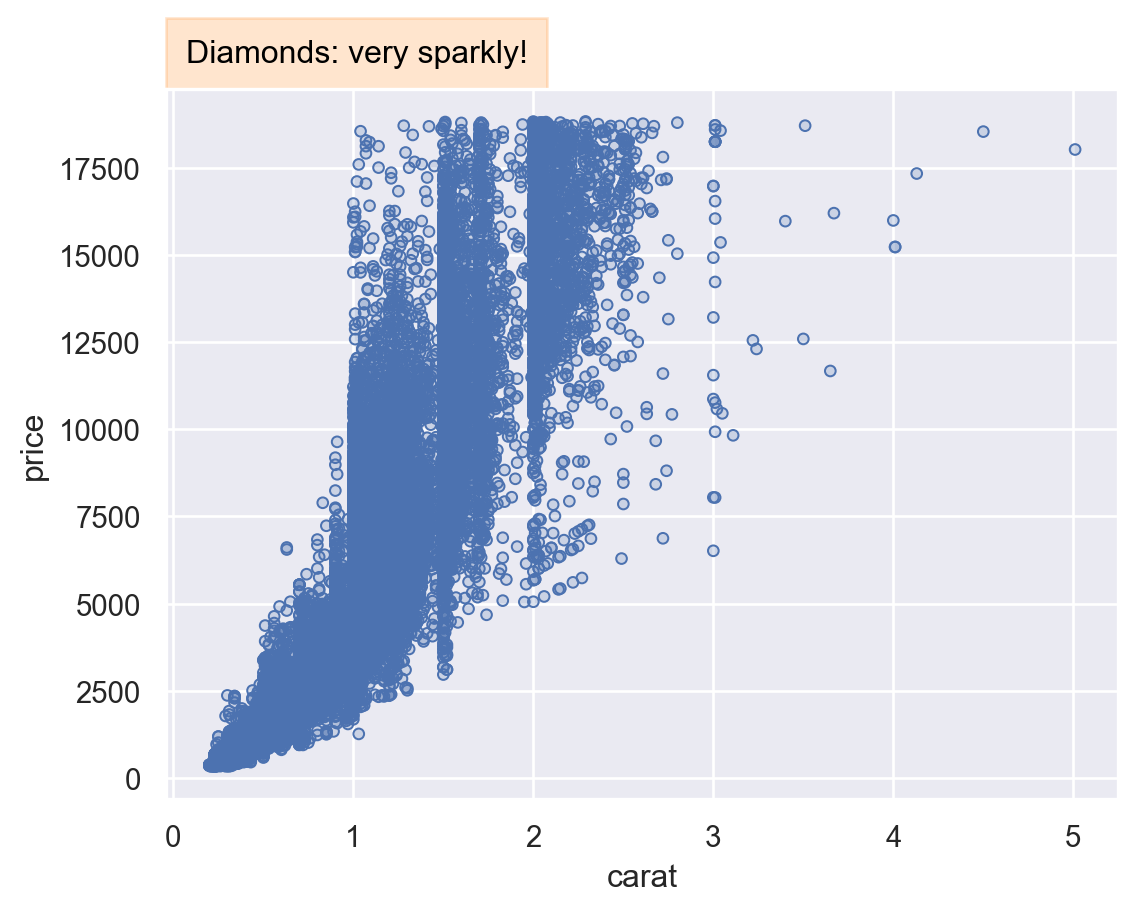Matplotlib 3.4 introduced the concept of `matplotlib.figure.Figure.subfigures()`, which make it easier to composite multiple arrangements of subplots. These can also be passed to `Plot.on()`,

```f = mpl.figure.Figure(figsize=(7, 4), dpi=100, layout="constrained")
sf1, sf2 = f.subfigures(1, 2)

p.on(sf1).plot()
(
so.Plot(diamonds, x="price")